## Writing Samples

### Articles at realpython.com

#### “Python’s map(): Processing Iterables Without a Loop”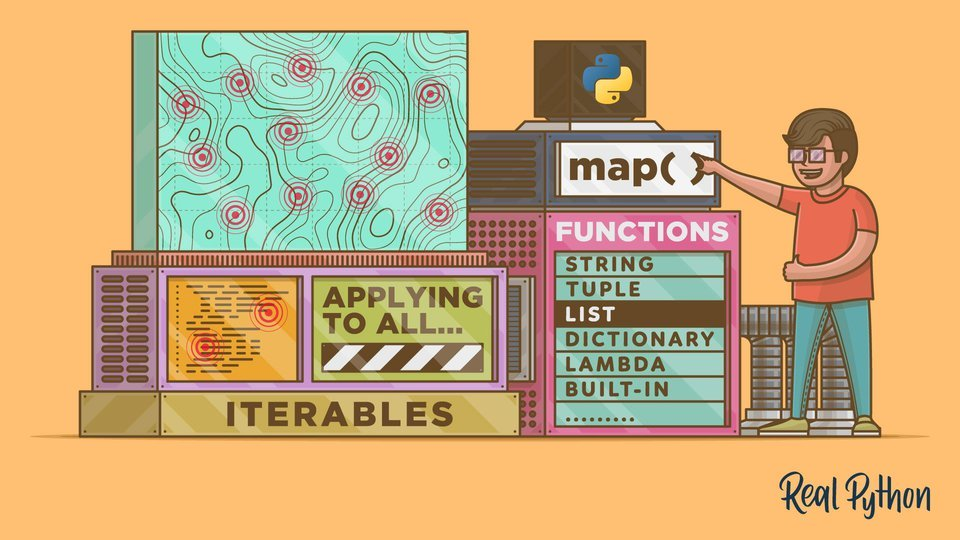In this step-by-step tutorial, you’ll learn how Python’s map() works and how to use it effectively in your programs. You’ll also learn how to use list comprehension and generator expressions to replace map() in a Pythonic and efficient way.

#### “The Python return Statement: Usage and Best Practices”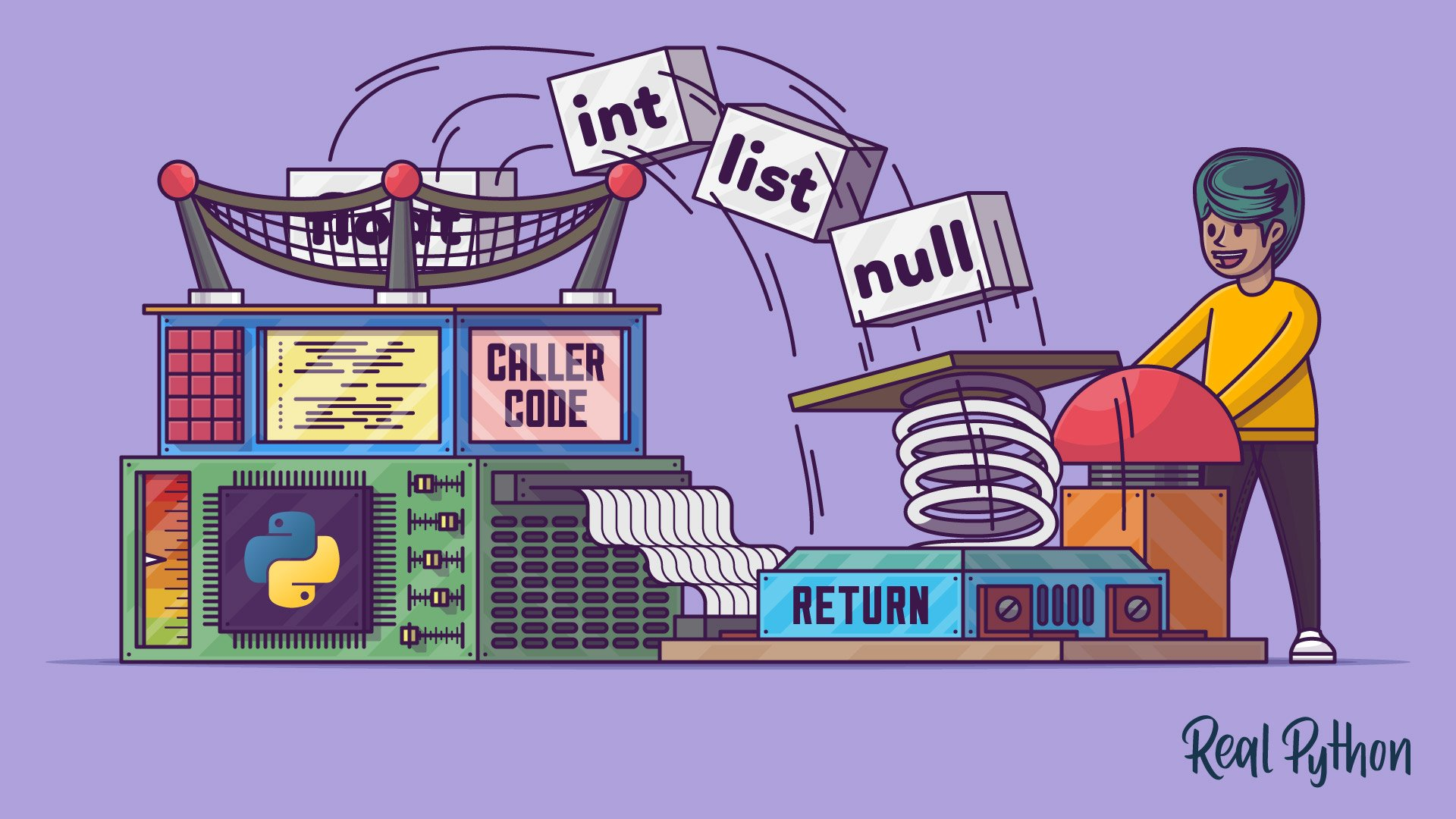In this step-by-step tutorial, you’ll learn how to use the Python return statement when writing functions. Additionally, you’ll cover some good programming practices related to the use of return. With this knowledge, you’ll be able to write readable, robust, and maintainable functions in Python.

#### “Python’s reduce(): From Functional to Pythonic Style”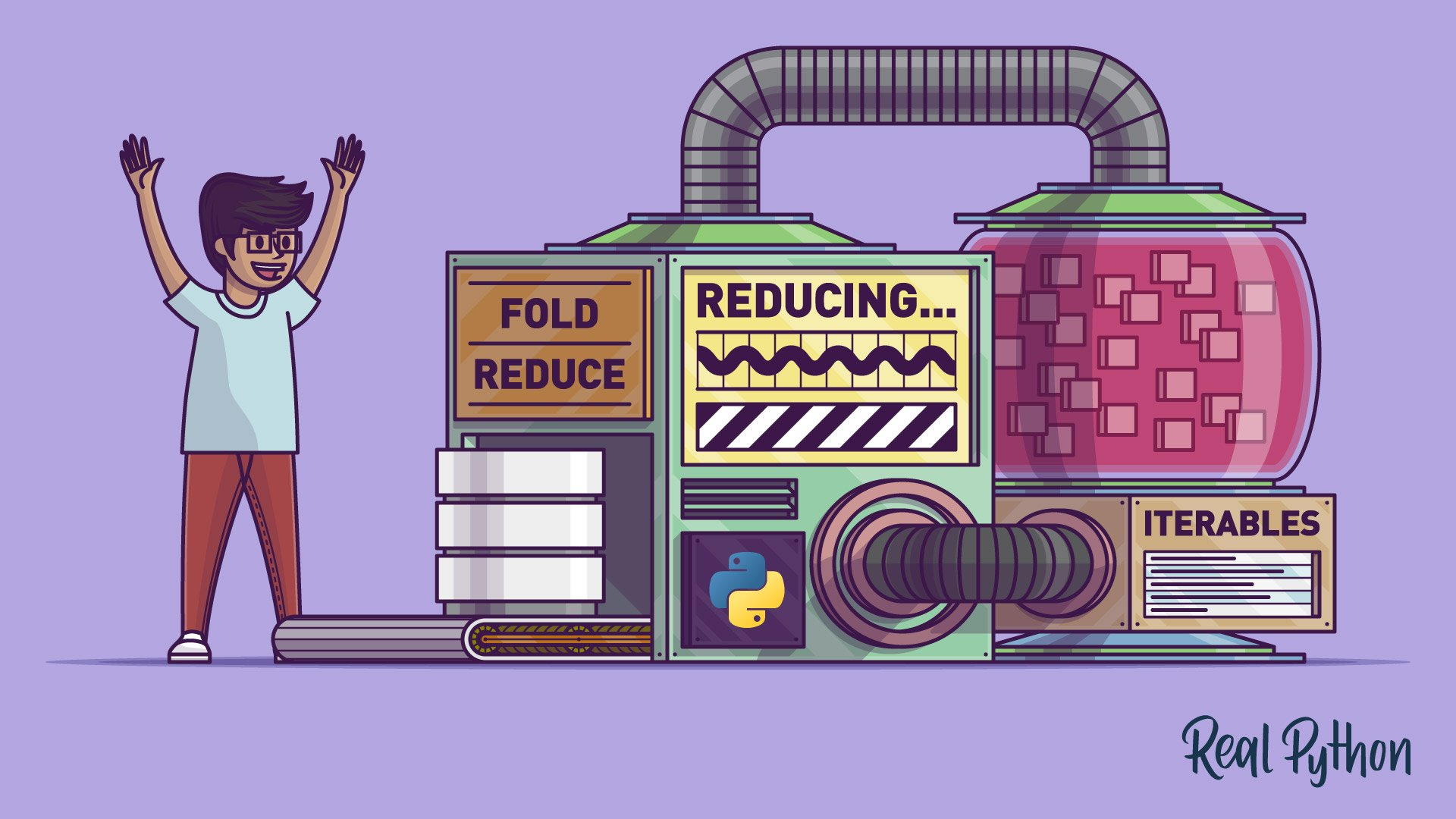In this step-by-step tutorial, you’ll learn how Python’s reduce() works and how to use it effectively in your programs. You’ll also learn some more modern, efficient, and Pythonic ways to gently replace reduce() in your programs.

#### “Python eval(): Evaluate Expressions Dynamically”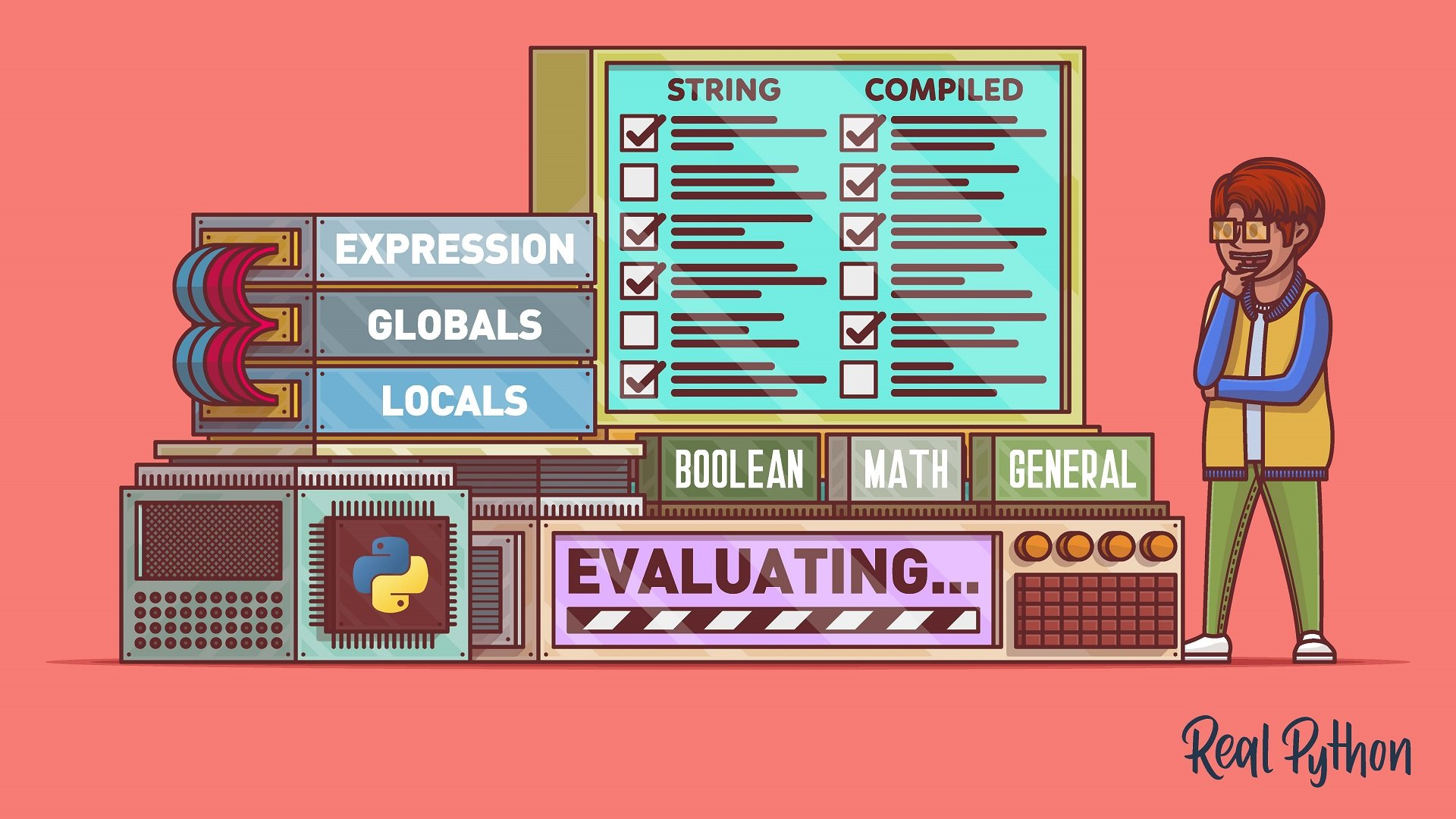In this step-by-step tutorial, you’ll learn how Python’s eval() works and how to use it effectively in your programs. Additionally, you’ll learn how to minimize the security risks associated to the use of eval().

#### “Python Scope & the LEGB Rule: Resolving Names in Your Code”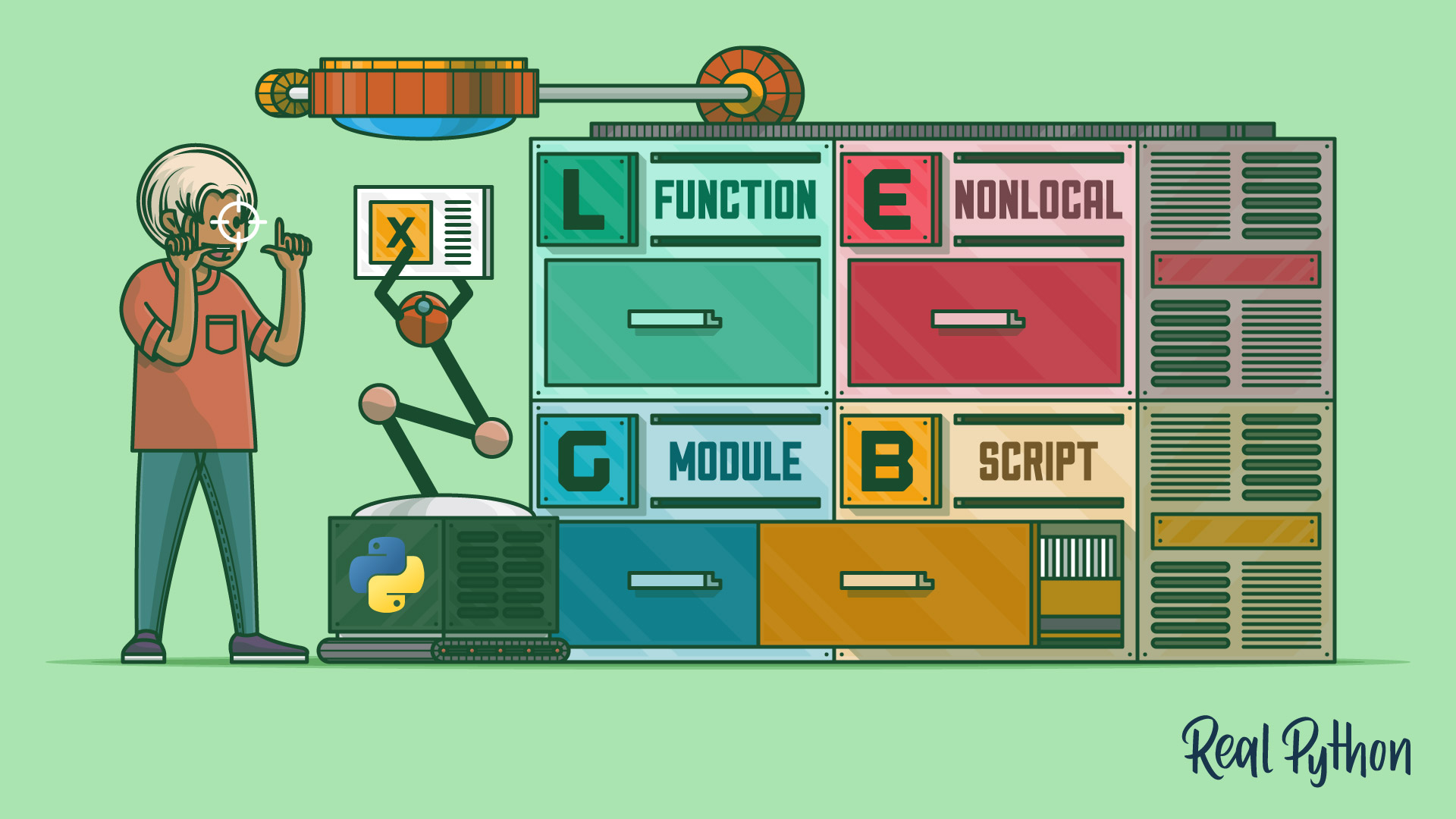In this step-by-step tutorial, you’ll learn what scopes are, how they work, and how to use them effectively to avoid name collisions in your code. Additionally, you’ll learn how to take advantage of a Python scope to write more maintainable and less buggy code.

#### “Using the Python defaultdict Type for Handling Missing Keys”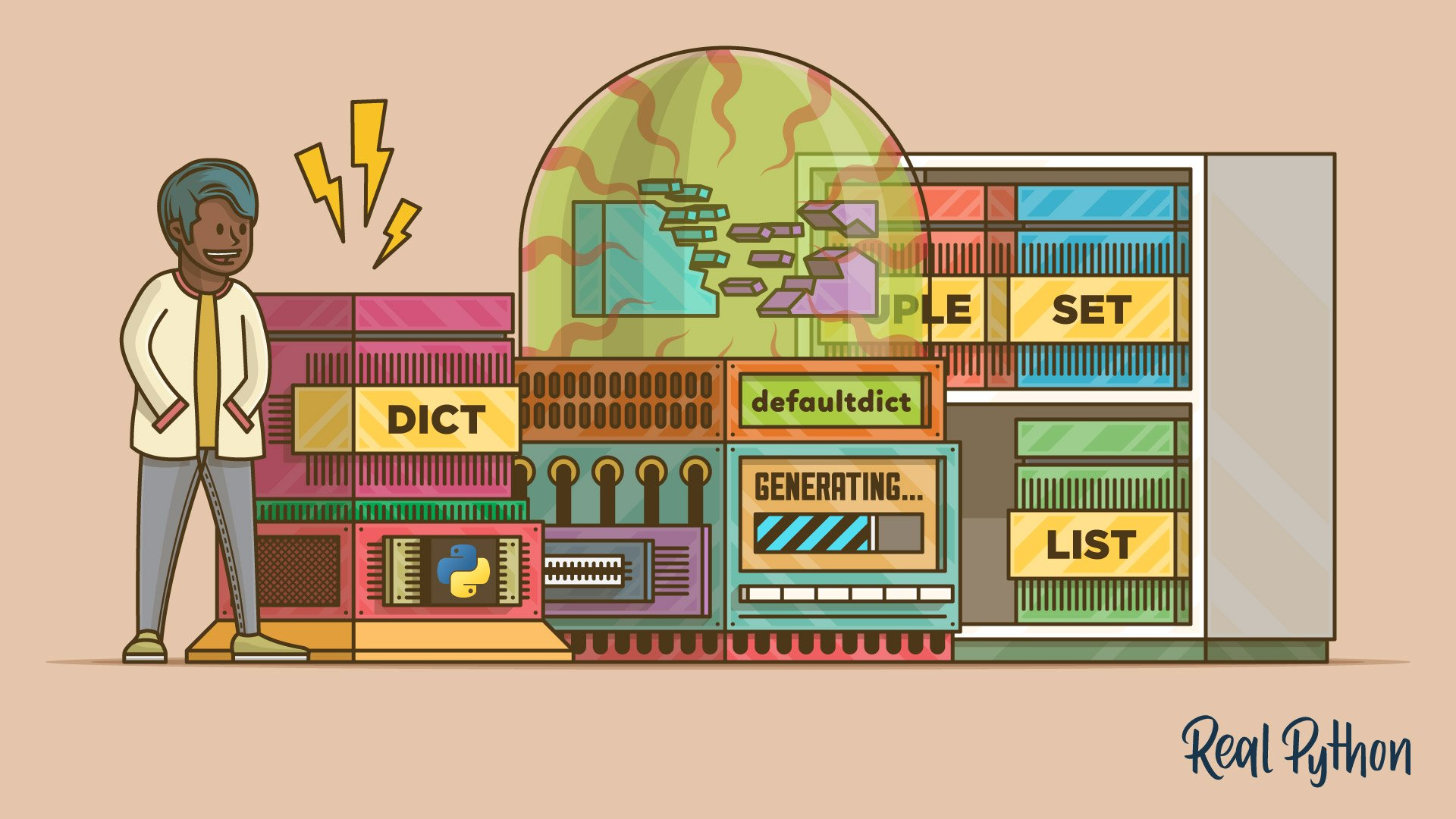In this step-by-step tutorial, you’ll learn how the Python defaultdict type works and how to use it for handling missing keys when you’re working with dictionaries. You’ll also learn how to use a defaultdict to solve problems like grouping or counting the items in a sequence or collection.

#### “Python and PyQt: Building a GUI Desktop Calculator”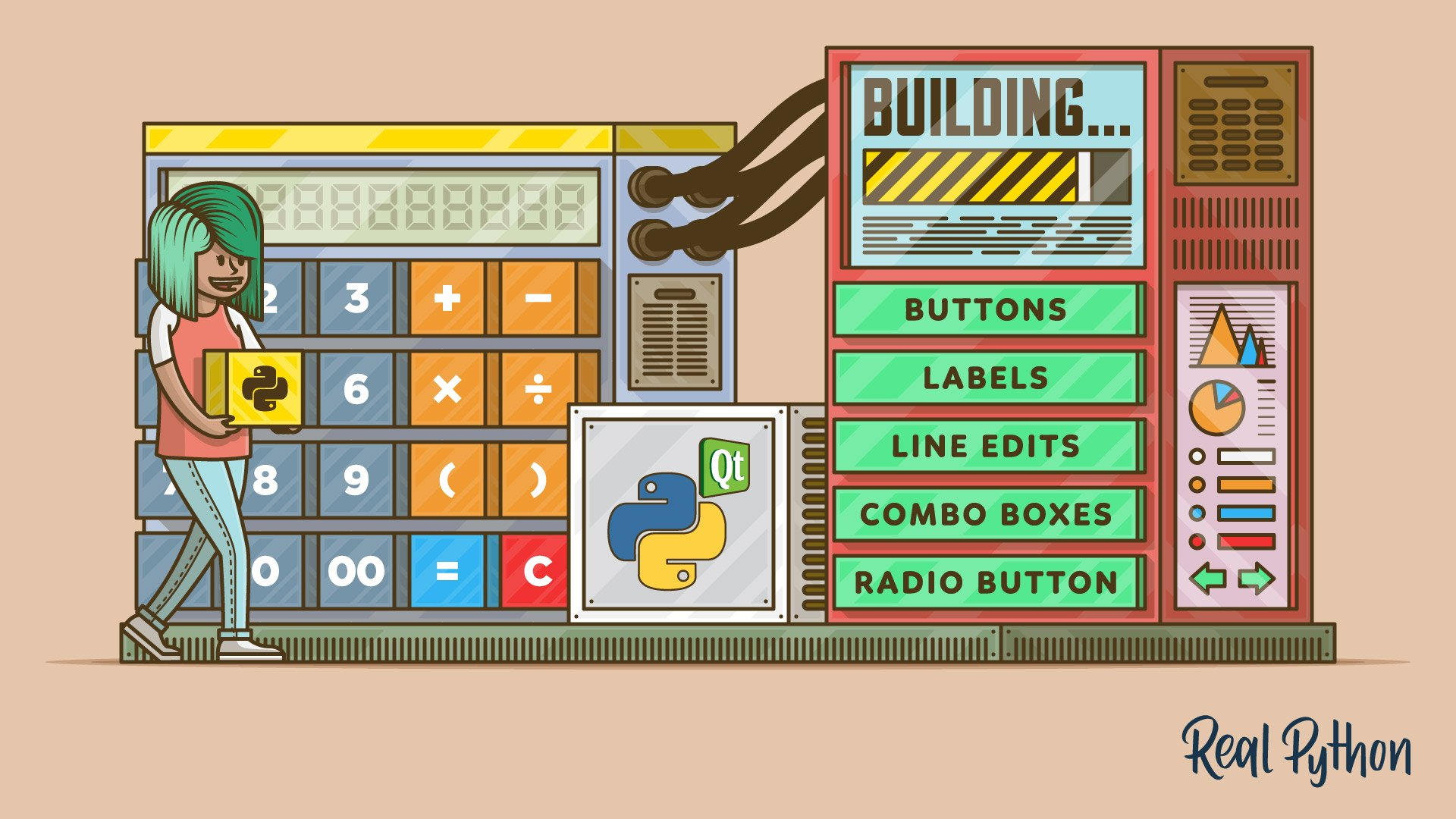In this step-by-step tutorial, you’ll learn how to create Graphical User Interface (GUI) applications with Python and PyQt. Once you’ve covered the basics, you’ll build a fully-functional desktop calculator that can respond to user events with concrete actions.

#### “Using the Python zip() Function for Parallel Iteration”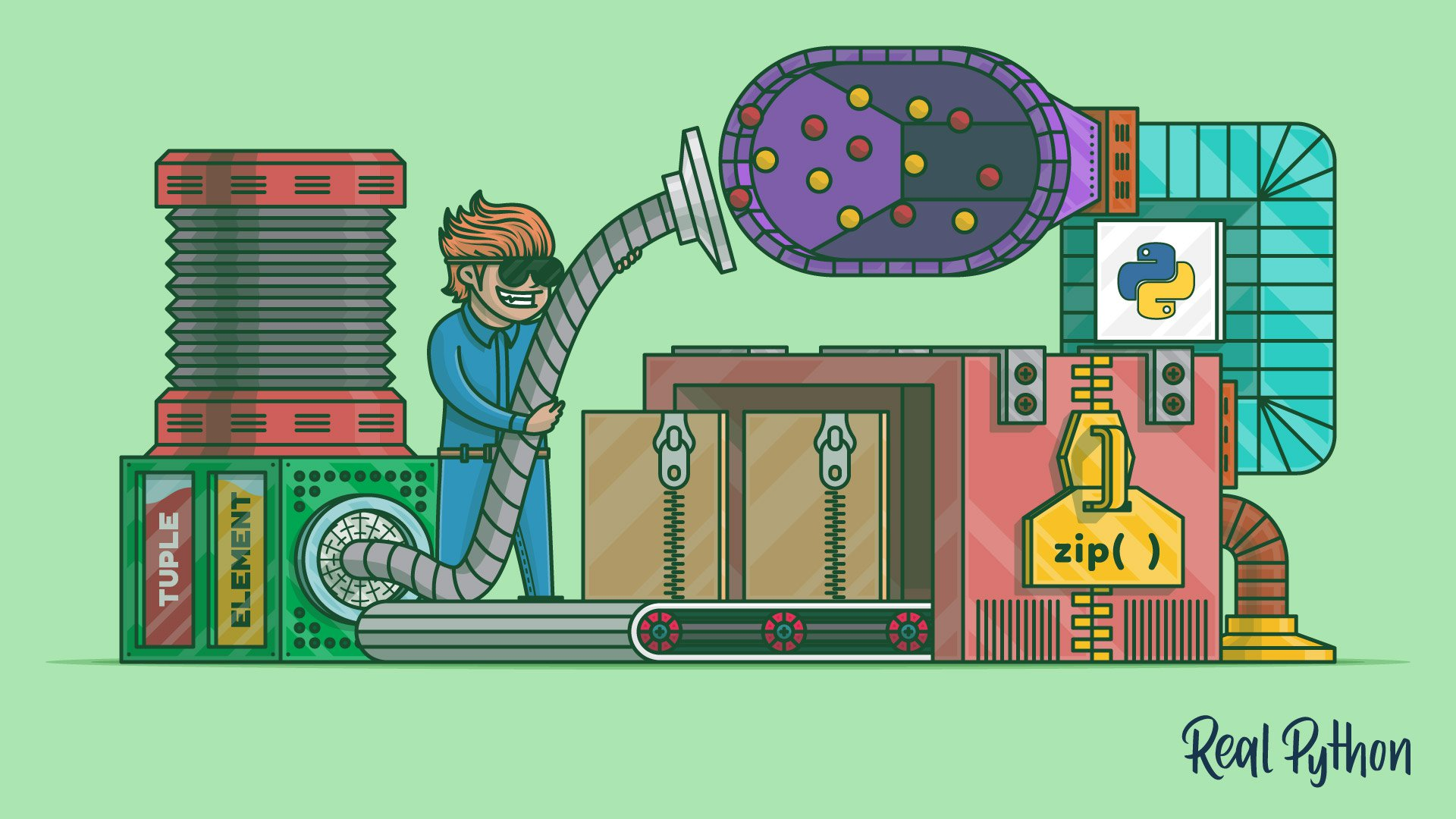In this step-by-step tutorial, you’ll learn how to use the Python zip() function to solve common programming problems. You’ll learn how to traverse multiple iterables in parallel and create dictionaries with just a few lines of code.

#### “How to Use the Python or Operator”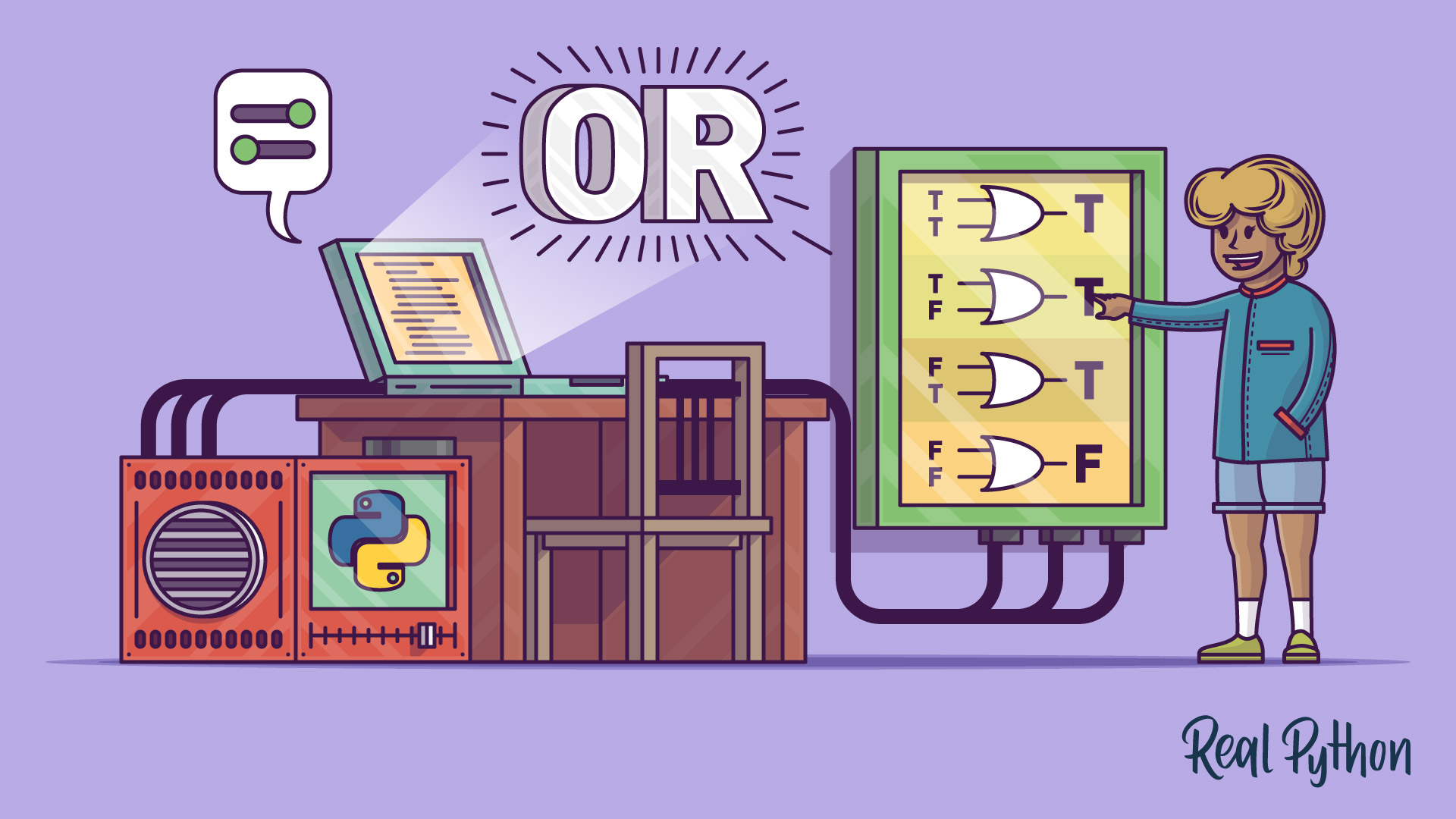In this step-by-step tutorial, you’ll learn about how the Python or operator works and how to use it. You’ll get to know its special features and see what kind of programming problems you can solve by using or in Python.

#### “How to Iterate Through a Dictionary in Python”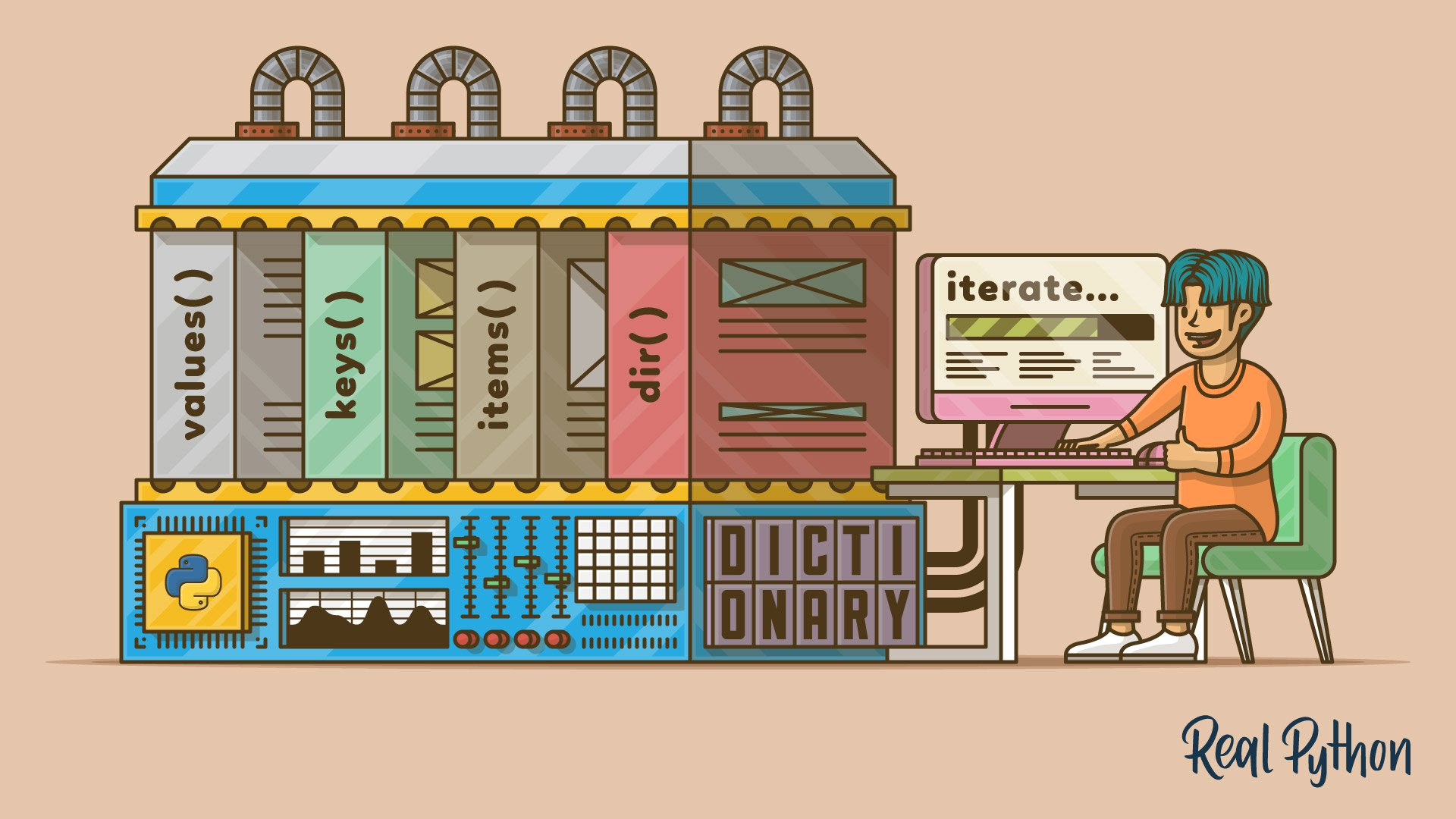In this step-by-step tutorial, you’ll take a deep dive into how to iterate through a dictionary in Python. Dictionaries are a fundamental data structure, and you’ll be able to solve a wide variety of programming problems by iterating through them.

#### “How to Run Your Python Scripts”This step-by-step tutorial will guide you through a series of ways to run Python scripts, depending on your environment, platform, needs, and skills as a programmer.

### Articles at stackabuse.com

#### “Calculating Variance and Standard Deviation in Python”In this step-by-step tutorial, you’ll learn how to calculate the variance and the standard deviation of a dataset in Python.

#### “Calculating Mean, Median and Mode in Python”In this step-by-step tutorial, you’ll learn how to calculate the mean, median, and mode of a sample of data in Python.

#### “Unpacking in Python: Beyond Parallel Assignment”In this step-by-step tutorial, you’ll learn how to perform iterable unpacking operations in Python.

#### “Formatting Strings with the Python Template Class”In this step-by-step tutorial, you’ll learn how to use the Python `Template` class. With `Templates`, we gain a heavily customizable interface for string substitution (or string interpolation).

### Articles at learnpyqt.com

#### “Creating Dialogs With Qt Designer”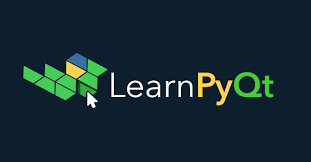In this step-by-step tutorial, you’ll learn how to use Qt Designer to build dialogs for your desktop applications.

#### “Laying Out Your GUIs With Qt Designer”In this step-by-step tutorial, you’ll learn how to to use Qt’s layouts with Qt Designer to build complex GUIs for your desktop applications.

## Open Source Projects Samples

VideoMorph: A user-friendly Video Converter based on FFMPEG and writen in Python/PyQt5.

Lubepy: A library that provides a set of basic calculations for machinery lubrication engineering. It’s designed to be use on field to perform fast calculations.

PyCalc: A sample calculator implemented using Python 3. The GUI is built using PyQt5, Tkinter, PySide2, and wxPython to show the flexibility of the Model-View-Controller (MVC) pattern. PyCalc is a sample project that extends the example I used in my Real Python tutorial: Python and PyQt: Building a GUI Desktop Calculator.

MathREPL: A math expression evaluator using Python eval() and the math module. MathREPL is a sample project based on the example used in the Real Python tutorial: Python eval(): Evaluate Expressions Dynamically.